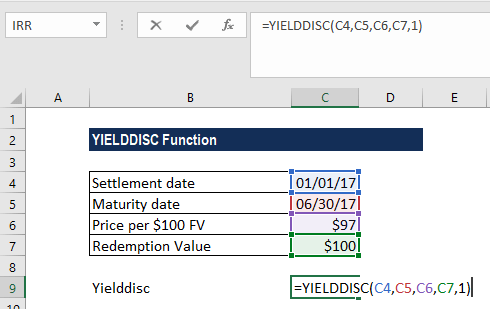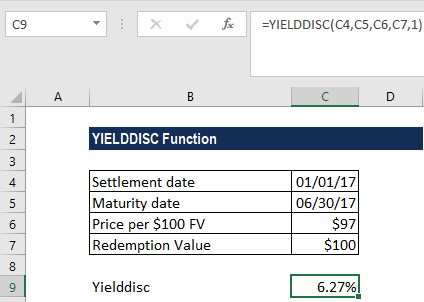# YIELDDISC Function

Calculates the annual yield for a discounted security

## What is the YIELDDISC Function?

The YIELDDISC function is categorized under Excel Financial functions. It will calculate the annual yield for a discounted security.

As a financial analyst, we often calculate the yield on a bond to determine the income that would be generated in a year. Yield is different from the rate of return, as the latter is the gain already earned, while yield is the prospective return. The YIELDDISC function is not the same as the YIELD function, as it calculates the yield on a discounted security.

### Formula

=YIELDDISC(settlement, maturity, pr, redemption, [basis])

The YIELDDISC function uses the following arguments:

1. Settlement (required argument) – This is the settlement date of the security. It is a date after the security is traded to the buyer – that is, after the issue date.
2. Maturity (required argument) – This is the maturity date of the security or the date when it expires.
3. Pr (required argument) – The price of the security per \$100 face value.
4. Redemption (required argument) – This is the redemption value per \$100 face value.
5. [basis] (optional argument) – Specifies the financial day count basis that is used by security. The possible values are:

BasisDay Count basis
0 or omittedUS(NASD) 30/360
1Actual/actual
2Actual/360
3Actual/365
4European 30/360

The settlement and maturity dates should be supplied to the YIELD function as either:

• References to cells containing dates
• Dates returned from formulas.

### How to use the YIELDDISC Function in Excel?

As a worksheet function, YIELDDISC can be entered as part of a formula in a cell of a worksheet. To understand the uses of the function, let’s consider an example:

#### Example

Suppose we are given the following data:

• Settlement date: 01/01/2017
• Maturity date: 06/30/2017
• Price per \$100 FV: \$97
• Redemption value: \$100
• Count basis: Actual/actual

We can find out the yield using the YIELDDISC function. The formula to use will be:We get the result below:This calculates the yield on the discounted security as 6.27%.

In the above example:

1. We used as the basis the actual/actual basis.
2. As recommended by Microsoft, the date arguments were entered as references to cells containing dates.

### A few notes about the YIELDDISC Function:

1. #NUM! error – Occurs when:
• The settlement date provided is greater than or equal to the maturity date.
• We provide invalid numbers for the rate, pr, redemption, frequency, or [basis] arguments. That is, if we provide rate < 0; pr ≤ 0; redemption ≤ 0; or [basis] as any number other than 0, 1, 2, 3, or 4.
1. #VALUE! error – Occurs when:
• Any of the arguments provided is non-numeric.
• The settlement and maturity dates provided are not valid dates.
2. The result from the Excel RATE function appears to be the value 0 or appears as a percentage but shows no decimal places. This problem is often due to the formatting of the cell containing the function. If this is the case, fix the problem by formatting the cell to show a percentage with decimal places.
3. Settlement, maturity, and basis are truncated to integers.
4. The settlement date is the date a buyer purchases a security such as a bond. The maturity date is the date when a security expires. For example, suppose a 30-year bond is issued on January 1, 2012, and is purchased by a buyer six months later. The issue date would be January 1, 2012, the settlement date would be July 1, 2012, and the maturity date would be January 1, 2042, which is 30 years after the January 1, 2012 issue date.

Thanks for reading CFI’s guide to the Excel YIELDDISC function. By taking the time to learn and master these functions, you’ll significantly speed up your financial modeling. To learn more, check out these additional CFI resources:

• Excel Functions for Finance